## J Gómez-Dans (UCL & NCEO)¶

j.gomez-dans@ucl.ac.uk### What is radiative transfer theory?¶

• The properties of the leaves and the canopy are combined
• For analysis, untangle response of the leaves and canopy.
• We want to understand the processes and properties that control sunlight being reflected by an e.g. canopy.
• RT tries to derive a physical description of the fate of photons as they are scattered and absorbed within a canopy.
• ... then we'll embed them in a canopy.

### Modelling leaf optical properties¶

• Photon hits a leaf, it can be
• absorbed ($A$),
• scattered ($R$), or
• transmitted ($T$).
• From energy conservation $A+R+T=1$
• Implicit dependence on wavelength ($\lambda$)

### How does a leaf look like?¶

#### Monocot¶#### Dicot¶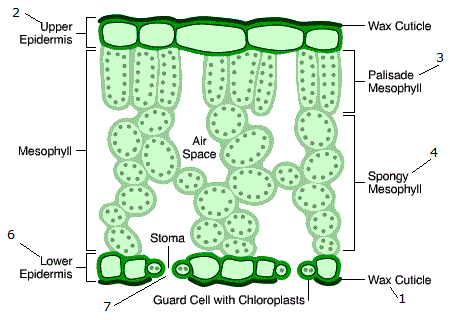### Leaf reflectance: directional aspects¶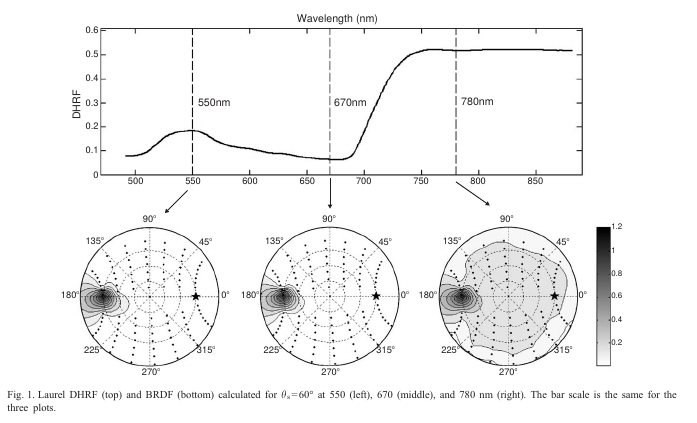• The wax cuticle is bright, and scatters incoming radiation with a strong directional signal in the specular direction, $R_s$.
• Specular response broadened by the leaf's surface microtopography.
• At interfaces between different materials, photons are scattered.
• Internal contribution $R_d$ is Lambertian (isotropic), the result of photons interacting with internal leaf structure and pigments.

### Leaf reflectance: spectral aspects¶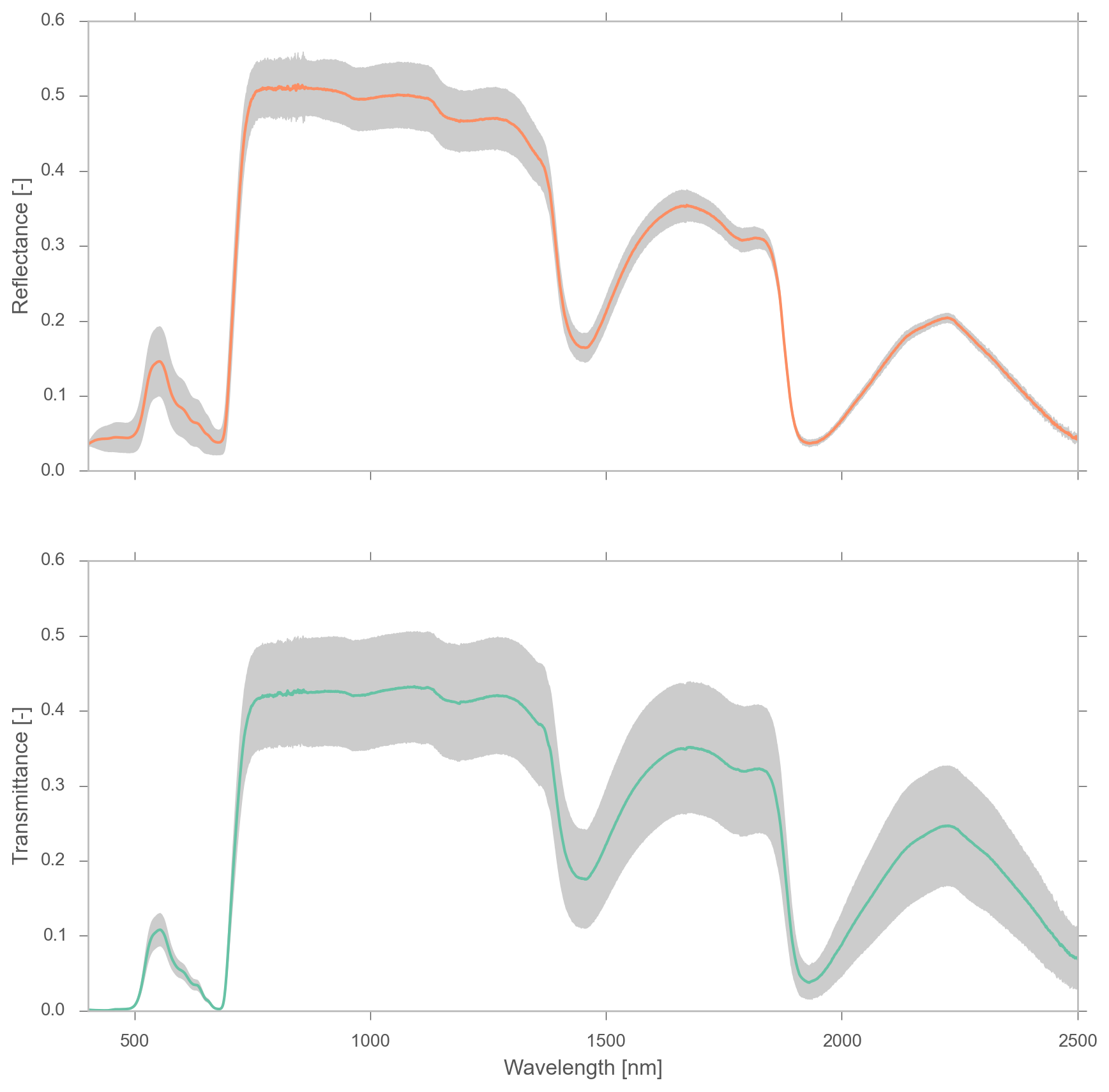Main spectral regions:

1. VIS $[400-700nm]$: absorption of radiation in green leaves. Chlorophyll, carotenoids, ..
2. NIR $[700-1100nm]$: multiple scattering due to air-cell wall interfaces,
3. SWIR $[>1100nm]$: mostly water absorption, note peaks at 1450, 1950 and 2500 nm.
• We will focus on the Lambertian effect
• This is a consequence of internal photon scattering
• therefore, it should tell us something about the internal structure and composition of the leaf.
• Although specular component is important, it is often neglected!

### A simple leaf model¶

• A monocot leaf looks like a slab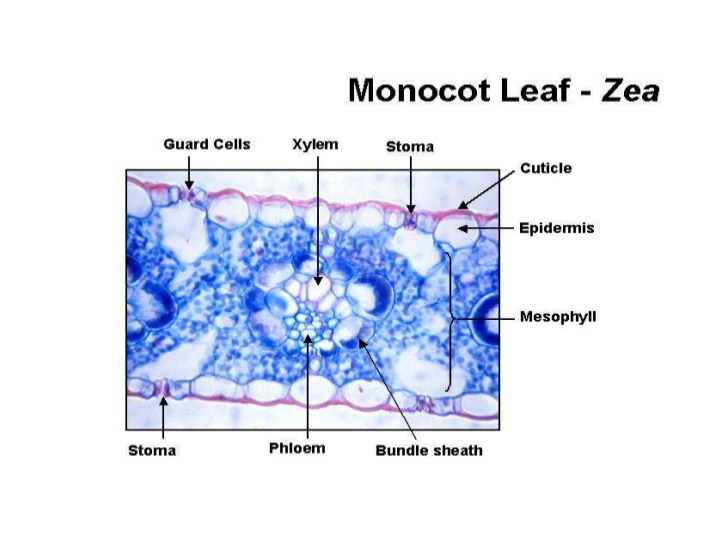• We can model it by considering the internal reflections of a bean inside a plate:• Solution to plate model given Airy in 1833, applied to leaves by Allen and others in 1970s
• We can write a fairly compact expression for the reflectance and transmittance of the plate that only depends on
• The index of reflection of the plate, $n$, and
• The absorption coefficient $k$.
• The model works surprisingly well for monocots, but fails for more complex leaves or senescent leaves.

### A multilayer leaf model¶

• Numerous photon scatter events take place at the air-cell boundaries within the leaf mesophyll
• So extend model to have a stack of plates separated by air interfaces to account for these interactions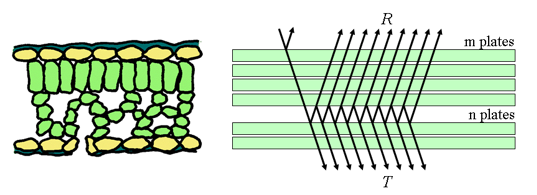• Problem solved by Stokes in 1862.
• Can calculate leaf reflectance and transmittance of $N$ layers as a function of a single layer ($R(1)$ and $T(1)$):
$$\frac{R(N)}{b^{N}-b^{-N}}=\frac{T(N)}{a-a^{-1}}=\frac{1}{ab^{N}-a^{-1}b^{-N}},$$

where

\begin{align} a &=\frac{1+R^{2}(1)-T^{2}(1) + \Delta}{2R(1)}\\ b &=\frac{1-R^{2}(1)+T^{2}(1) + \Delta}{2T(1)}\\ \Delta &= \sqrt { (T^{2}(1)-R^{2}(1)-1)-4R^{2}(1)}. \end{align}
• Can make number of layers a real number not an integer (e.g. 1.3 layers)
• $\Rightarrow$ PROSPECT
• PROSPECT predicts leaf reflectance and transmittance as a function of
• $N$ (the number of layers $\sim \left[1.5-2.5\right]$, a sort of internal complexity of the leaf parameter)
• Calculates $k$ from the concentrations of
• Chlorophyll a+b
• Leaf water
• "Brown pigment"
• "Dry matter"
• Carotenoids

#### Chlorophyll a+b specific absorption¶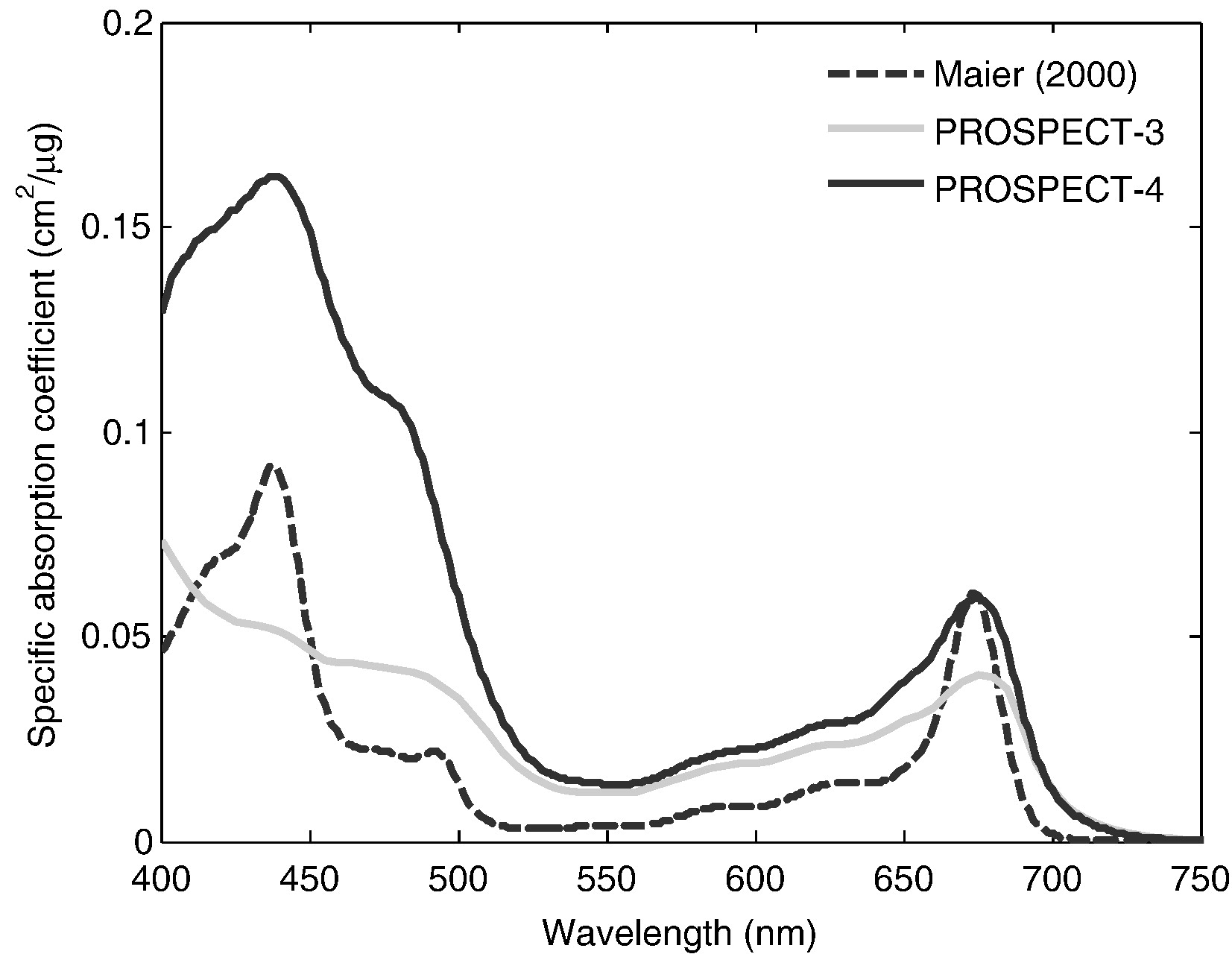#### Carotenoids specfic absorption¶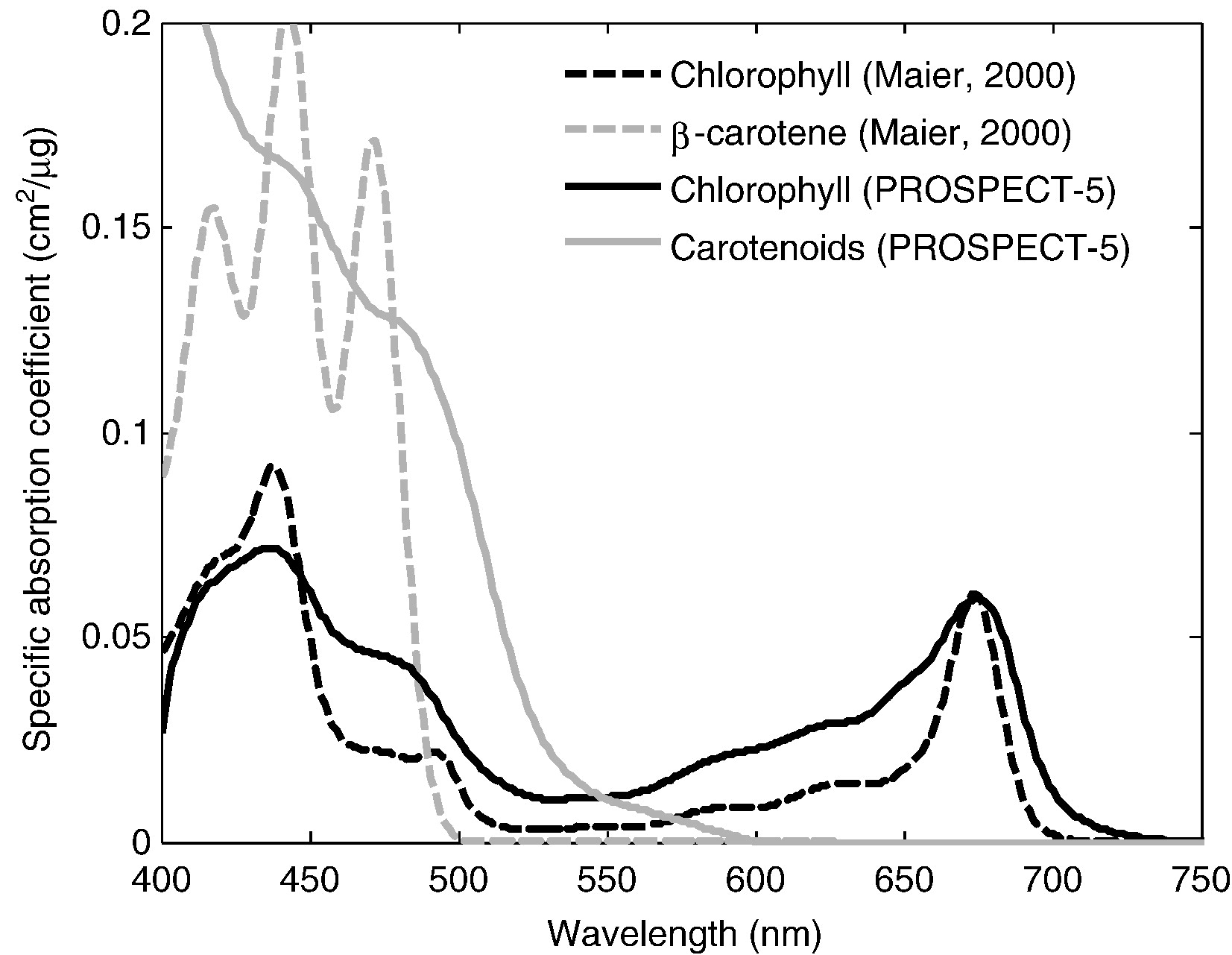#### Dry matter specific absorption¶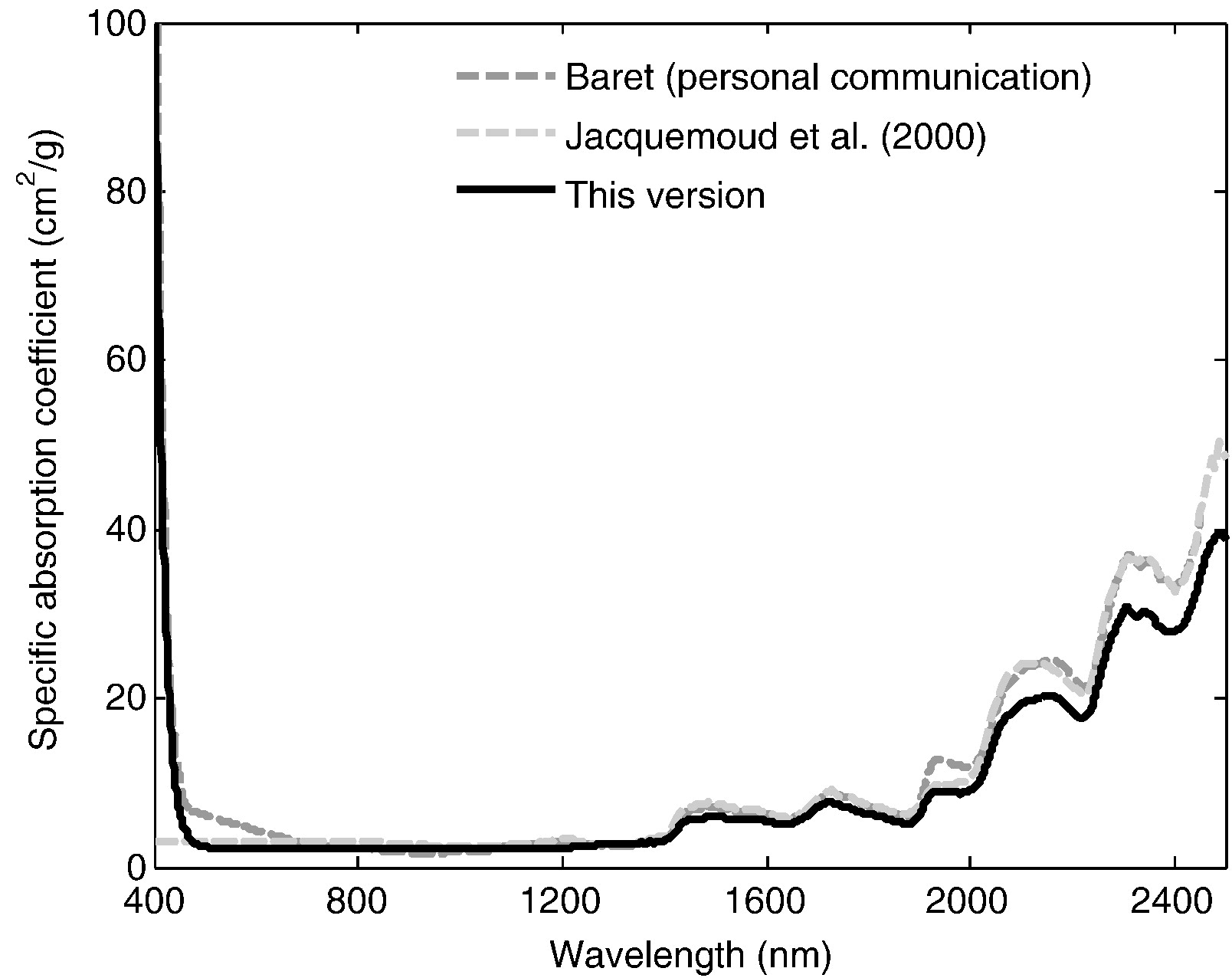#### Water specific absorption¶• Cellulose and lignine as well as proteins have particular absorption fingerprints
• However, absorption coincides with water absorption
• Hence these responses lumped into "Dry matter".

## Recap¶

• Leaf BRDF
• Directional component due to surface specular reflection
• Lambertian component due to internal interactions
• Leaves can be modelled as stacks of plates
• Parsimonious model with a handful of parameters
• PROSPECT:
• N layers ($N\in R$)
• Chlorophyll concentration $(\mu gcm^{-2})$
• Carotenoids $(\mu gcm^{-2})$
• Equivalent water thickness $(cm)$)
• Dry matter $(gcm^{-2})$